# Area Under the Curve and Integrals in AP Calculus: Help and Review Chapter Exam

Exam Instructions:

Choose your answers to the questions and click 'Next' to see the next set of questions. You can skip questions if you would like and come back to them later with the yellow "Go To First Skipped Question" button. When you have completed the practice exam, a green submit button will appear. Click it to see your results. Good luck!

### Page 1

#### Question 1 1. What is the area under the curve below using a single trapezoid?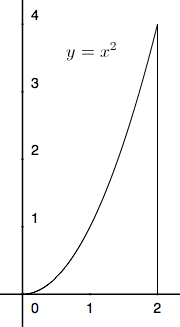#### Question 2 2. Given f(x) on the graph, what does z equal?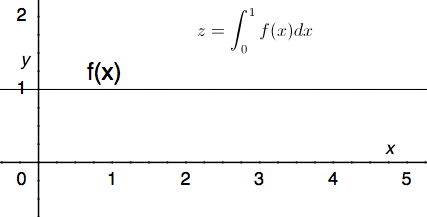#### Question 3 3. Which integral below is equal to the following integral?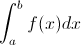#### Question 4 4. What is the equation for the length of the curve following y=f(x) between x=a and x=b given f(x) below?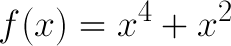#### Question 5 5. Which of the following represents the area of the first slice under the curve f(x) between x = 0 and x = 2 when divided into 4 slices?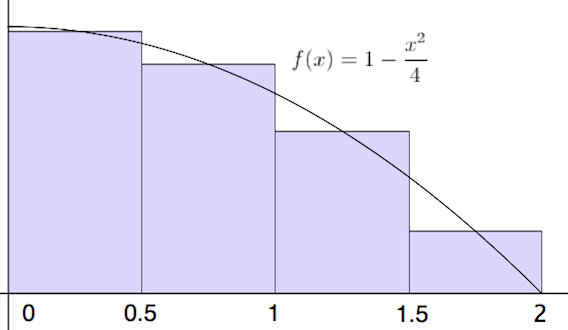### Page 2

#### Question 6 6. Which of the following can NOT be a solution for the integral below?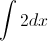#### Question 7 7. What is the one term left-sided Riemann Sum approximation for the integral below?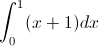#### Question 8 8. In the following equation, g(s) is the _____.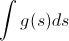#### Question 10 10. What is the one-term left-side Riemann sum for y=f(x) from 0 to 2 for the graph below?### Page 3

#### Question 11 11. The following integral can be re-written as: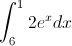#### Question 12 12. In the following integral, g(x) is defined as which of the following?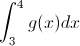#### Question 13 13. Given a continuous function f(x) that satisfies the equation below, which of the following is true, according to the average value theorem?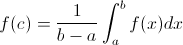#### Question 14 14. Which integral would be evaluated using the following expression (given that F'(x) = f(x))?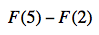#### Question 15 15. What is the two term left-sided Riemann Sum approximation for the integral below?### Page 4

#### Question 16 16. Use three trapezoids to find the area under the curve shown below between x=0 and x=3.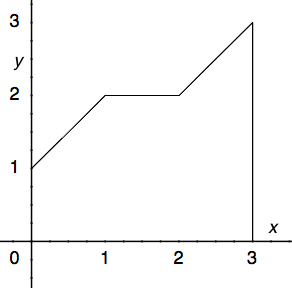#### Question 17 17. Which of the following integrals represents the area under the graph below?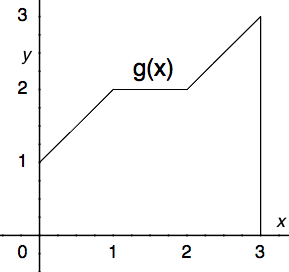#### Question 18 18. Which of the following represents a four-term right-handed Riemann sum for y=f(x)?#### Question 19 19. Evaluate the following.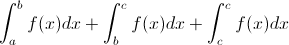#### Question 20 20. What is the two-term left-side Riemann sum for y between 0 and 2?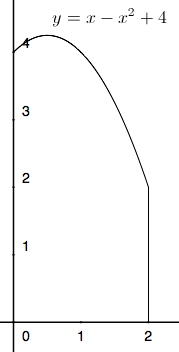### Page 5

#### Question 22 22. Which of the following represents a left-sided Riemann sum of the following integral with n = 4?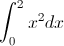#### Question 23 23. Which of the following integrals is equal to the following summation over the continuous region x=a to x=b?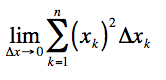#### Question 24 24. Given f(x) on the graph below, which of the following integrals will equal 0?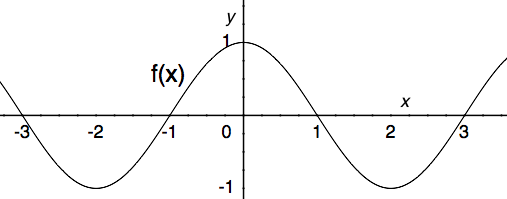#### Question 25 25. Estimate the area beneath the curve f(x) using the two term Riemann sum as shown below.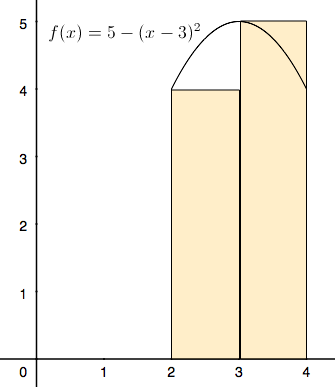### Page 6

#### Question 26 26. Use the fundamental theorem of calculus to evaluate the following integral.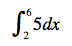#### Question 27 27. In the following integral, 4 is defined as which of the following?#### Question 29 29. Using the single term Riemann sum as shown, what is the area beneath the curve f(x) between 0 and two pi?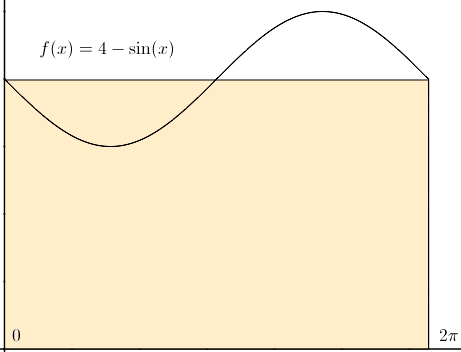#### Question 30 30. In the following equation, s is the _____.#### Area Under the Curve and Integrals in AP Calculus: Help and Review Chapter Exam Instructions

Choose your answers to the questions and click 'Next' to see the next set of questions. You can skip questions if you would like and come back to them later with the yellow "Go To First Skipped Question" button. When you have completed the practice exam, a green submit button will appear. Click it to see your results. Good luck!

Support